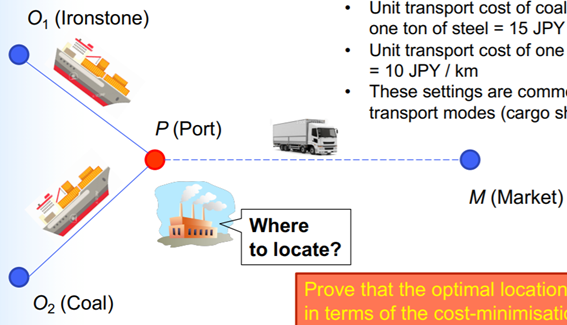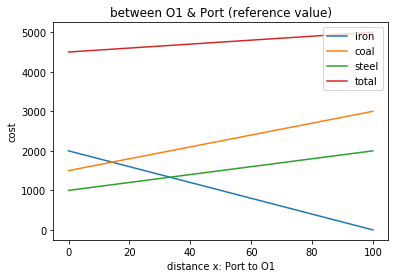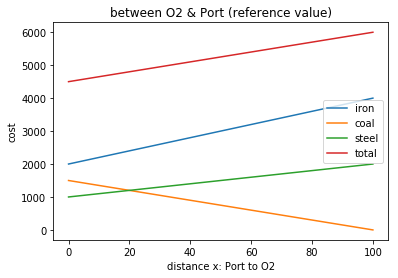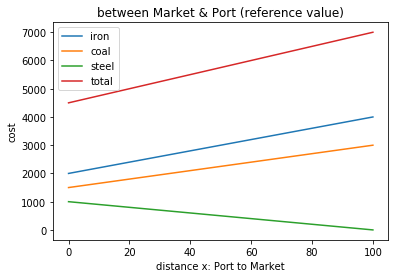# Transport Econ.

Python is my boom now since I use again as research in university.

### assignment issuein transport "economics" lecture in TokyoTech
This is lecture slide, So I show you it as possible as I can.

### code

```# -*- coding: utf-8 -*-
"""
Created on Sun Jan 26 14:34:11 2020

@author: aoki
"""
#config
import numpy as np
import matplotlib.pyplot as plt

where=['O1','O2','Market']
x=np.linspace(0,100)#100 is reference value to draw graph
#%%loop
for i in where:
#initialize
dummy=[1,1,1]
total_cost=0
#case
for j in range(len(where)):
if i==where[j]:
dummy[j]=-1
#%%cal (100 is reference value to draw graph too
cost_iron= 20*(100+x*dummy)
cost_coal= 15*(100+x*dummy)
cost_steel=10*(100+x*dummy)

total_cost=cost_iron+cost_coal+cost_steel
#%%output as graph
plt.plot(x,cost_iron,label='iron')
plt.plot(x,cost_coal,label='coal')
plt.plot(x,cost_steel,label='steel')
plt.plot(x,total_cost,label='total')
plt.legend()
plt.title('between '+i+' & Port (reference value)')
plt.xlabel('distance x: Port to '+i)
plt.ylabel('cost')
plt.show()
```

### outputs as a result### summary

The factory should be constructed just (near) a Port.
This conclusion is near my sense and measured in fact.